#In the early days when we were growing 2D structures and doing a few 3D plots not in the CAD domain, we investigated design growth under a static load.

## Principle

The core algorithm followed this simple principle:

Given a set of growth functions and design genes, for each shoot increase the diameter of the shoot if the local maximum stress is greater than total maximum stress, otherwise grow in length and angle. Simply put, grow bigger/thicker if local stress is too high and grow longer if local stress is within an acceptable value.

### Implementation

Lets break down the process step by step.

#### Step 1

Given the example in figure 2, a seed is first planted with a set of design genes that include a length delta and diameter delta. The seed is grown by these delta values in the first growth step. As the design system loops back around to the next growth step, we start to check whether to grow bigger or longer.

#### Step 2

Initially, we use assume there is only one section/part in the system, using the bending moment equation we can calculate whether the stress in this section is bigger or smaller than the overall stress of the shoot (Figure 3).

#### Step 3

Now inside the loop of the growth function we are checking if the stress is larger than the stress of the system, all to determine whether to grow in diameter. Remember this is still only for the first section.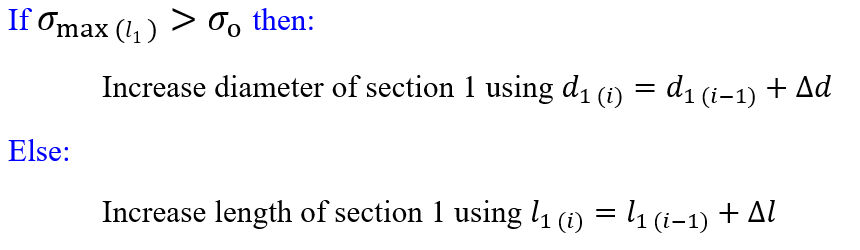Figure 4 – Section of code that checks the stress value in that section to determine what growth function shall occur

#### Step 4

The system continues looping through Steps 2-3 until a new section emerges. At this point, we did not know exactly how or why a new “section/part” would emerge, we were questioning why exactly does a cell stop growing and start dividing? Remember this is only the initial idea and testing of our concept! Okay back to the problem at hand..

So eventually a new section emerges, for this to occur, we have assumed once section 1 reaches a defined length we create a new section and stop checking against section 1.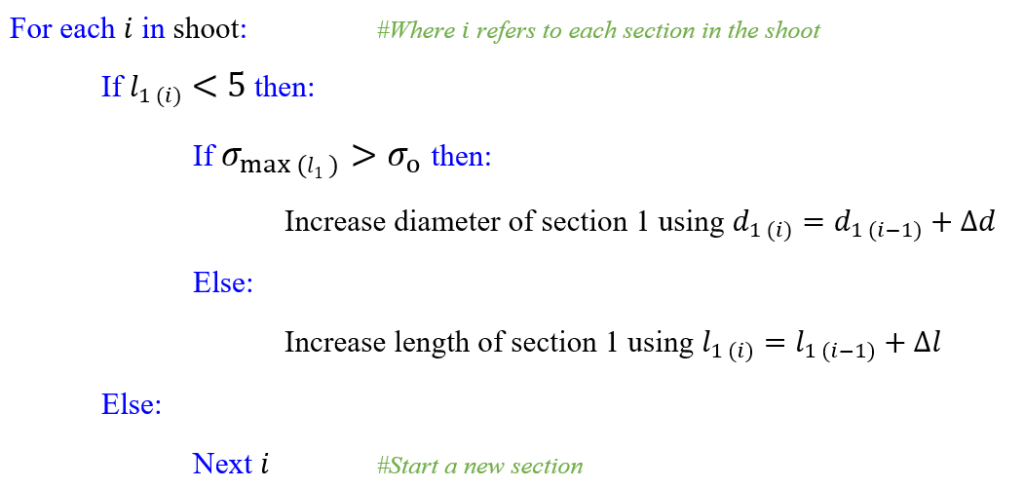Figure 5 – Update of code that creates a new section in the shoot based on the length of the current section

#### Step 5

Similarly to steps 2 & 3, we now check the stress in part/section 2 against the system maximum. However, if the local stress is now greater than the maximum, we grow bigger in BOTH section 1 and 2.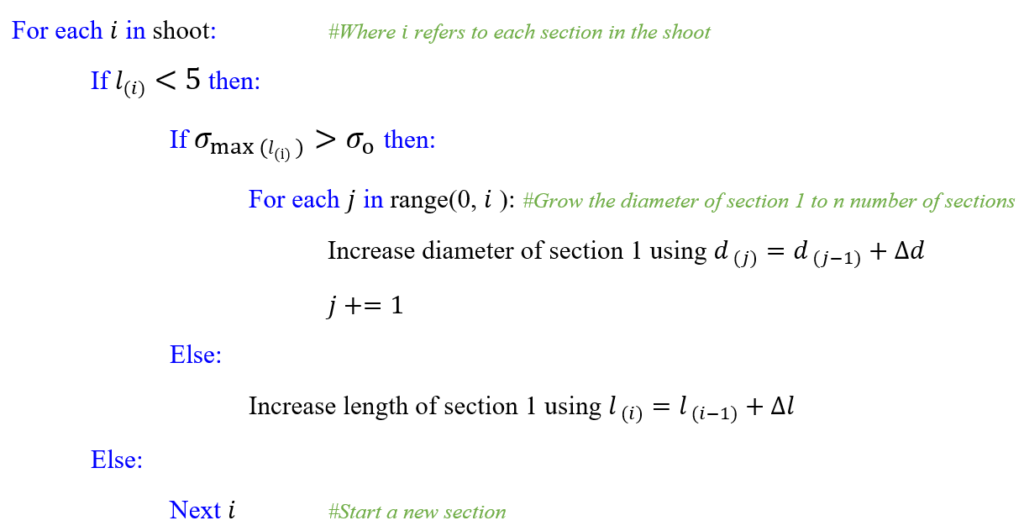Figure 6 – Further updates enable the code to grow the diameter of each section in the shoot based on the stress value of its current section

#### Step 6

Again, we iterate steps 2-5 growing another section and increasing in size based on the stress.

However at the end of the design cycle in this iteration, when the length of section/part 3 is greater than five instead of just one new section emerging we split the section in to two. We referred to this phenomenon as ‘branching’ or if you have read more of our recent works ‘budding’, either way we split this section and then iterate through the same steps as above.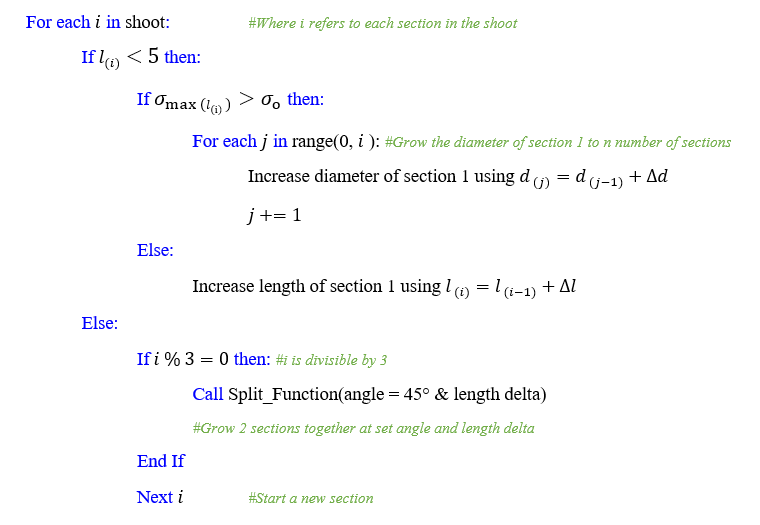Figure 7 – A check at the end of the growth is included to now split the next section into 2 if the current section is divisible by 3

#### Step 7

Eventually the design cycle has to come to an end. Ideally there would be a natural stopping point but unfortunately we haven’t quite figured out where that is at this point. So how do we stop the loop and the growth process? Well, actually, we simply tell the design system to stop growing at a particular growth step. So at growth step 25 we stop or at growth step 50 we stop, it is a hard coded number defined by us.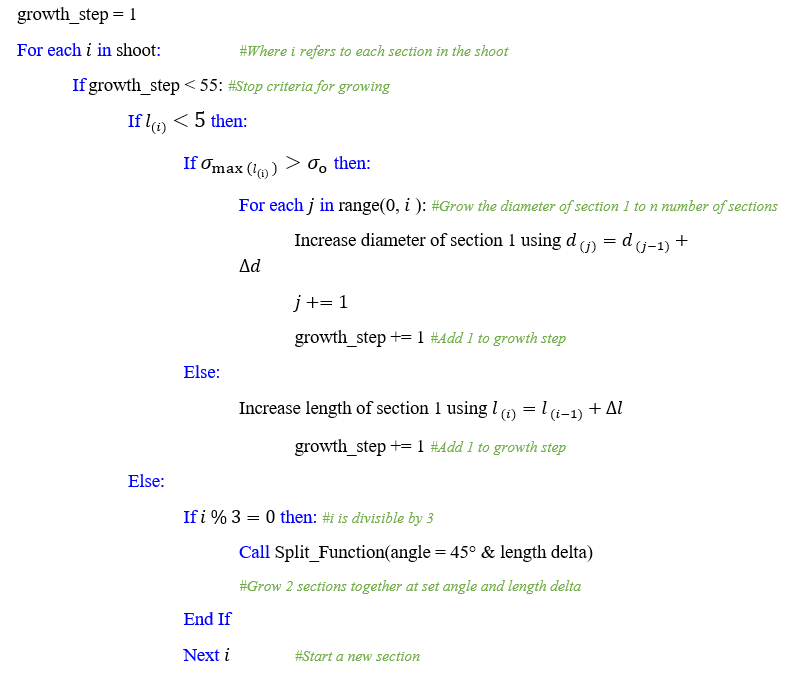Figure 8 – Design loop with the inclusion of a stop criteria, stopping at 55 growth steps

We know this is not very eloquent but it was suitable for testing purposes. There were a few assumptions made throughout this experiment, they are listed at the end of this article, but in the meantime lets review the results.

## Results

Below are a number of plots at the different stages of the growth cycle outlined above. Note how each section/part continues to grow bigger i.e. diameter, even when a new section has emerged. The size of the last plot in figure 10 in the bottom right is much bigger than that of the first plot in the top left of figure 9, section 1 in particular.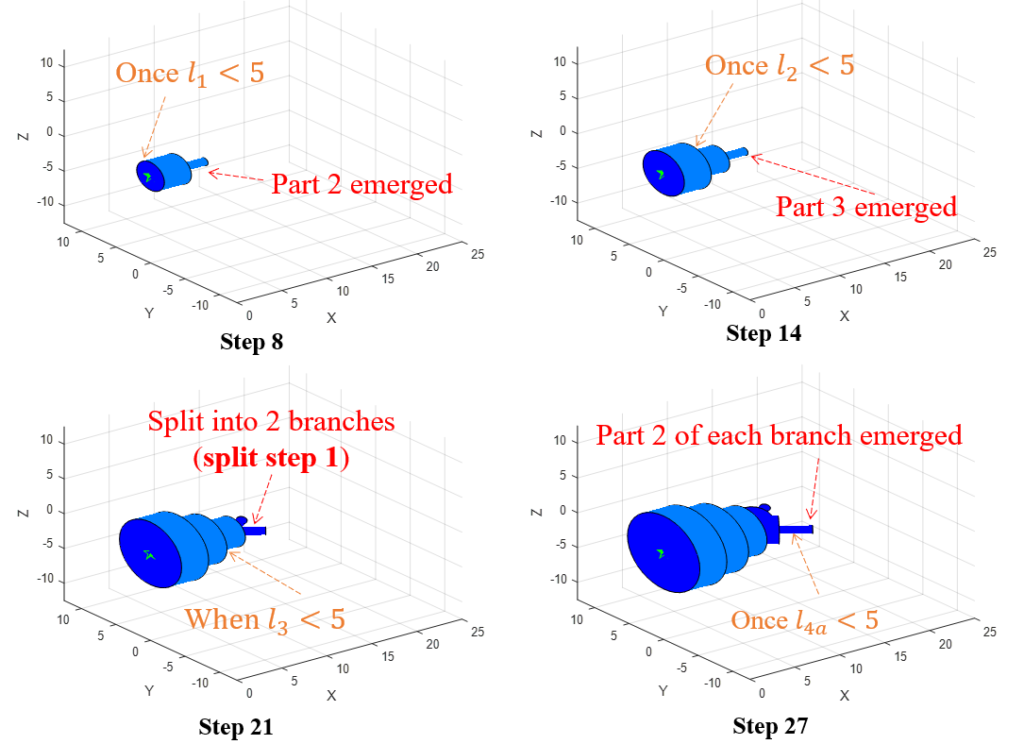Figure 9 – Four plots of growing & splitting the shoot in length and diameter up to the 27th growth step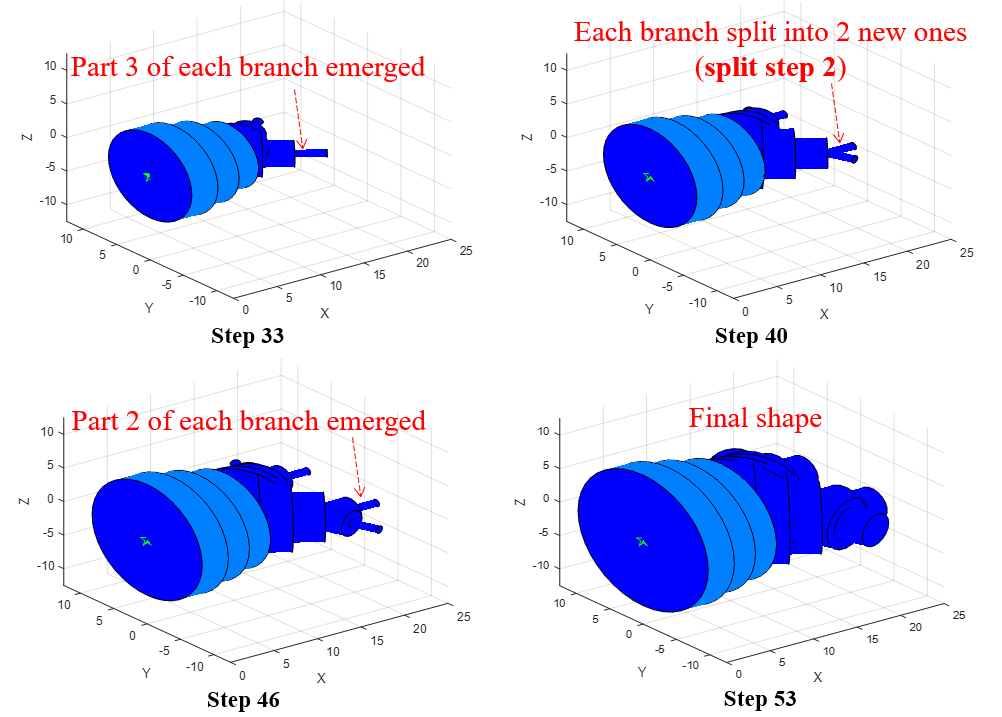Figure 10 – Further growth plots, showing how the design system continually grows along the entire shoot and eventually splits again

## Assumptions in System

We are aware that to test this theory we had to make a few assumptions, they are listed below.

1.Grow until a set number of growth steps is reached

2.Emerge a new part/section when current section reaches a certain length

3.Split the shoot into branches when the section is at a certain number

## Questions for Biohaviour to Answer

Arising from this particular research piece there are a few critical questions for the team at Biohaviour to answer, they are listed below.

1.How do nature systems naturally evolve from growing to dividing and growing?

2.Why do biological systems split and change direction? How does this occur? How is the angle determined?